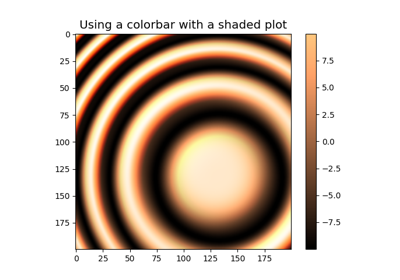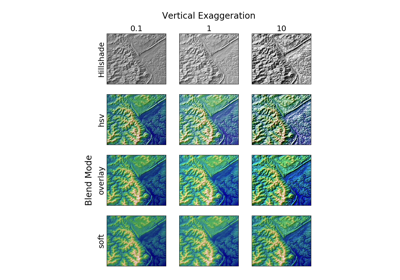Travis-CI:# matplotlib.colors.LightSource¶

class `matplotlib.colors.``LightSource`(azdeg=315, altdeg=45, hsv_min_val=0, hsv_max_val=1, hsv_min_sat=1, hsv_max_sat=0)

Create a light source coming from the specified azimuth and elevation. Angles are in degrees, with the azimuth measured clockwise from north and elevation up from the zero plane of the surface.

The `shade()` is used to produce “shaded” rgb values for a data array. `shade_rgb()` can be used to combine an rgb image with The `shade_rgb()` The `hillshade()` produces an illumination map of a surface.

Specify the azimuth (measured clockwise from south) and altitude (measured up from the plane of the surface) of the light source in degrees.

Parameters: azdeg : number, optional The azimuth (0-360, degrees clockwise from North) of the light source. Defaults to 315 degrees (from the northwest). altdeg : number, optional The altitude (0-90, degrees up from horizontal) of the light source. Defaults to 45 degrees from horizontal.

Notes

For backwards compatibility, the parameters hsv_min_val, hsv_max_val, hsv_min_sat, and hsv_max_sat may be supplied at initialization as well. However, these parameters will only be used if “blend_mode=’hsv’” is passed into `shade()` or `shade_rgb()`. See the documentation for `blend_hsv()` for more details.

`blend_hsv`(rgb, intensity, hsv_max_sat=None, hsv_max_val=None, hsv_min_val=None, hsv_min_sat=None)

Take the input data array, convert to HSV values in the given colormap, then adjust those color values to give the impression of a shaded relief map with a specified light source. RGBA values are returned, which can then be used to plot the shaded image with imshow.

The color of the resulting image will be darkened by moving the (s,v) values (in hsv colorspace) toward (hsv_min_sat, hsv_min_val) in the shaded regions, or lightened by sliding (s,v) toward (hsv_max_sat hsv_max_val) in regions that are illuminated. The default extremes are chose so that completely shaded points are nearly black (s = 1, v = 0) and completely illuminated points are nearly white (s = 0, v = 1).

Parameters: rgb : ndarray An MxNx3 RGB array of floats ranging from 0 to 1 (color image). intensity : ndarray An MxNx1 array of floats ranging from 0 to 1 (grayscale image). hsv_max_sat : number, optional The maximum saturation value that the intensity map can shift the output image to. Defaults to 1. hsv_min_sat : number, optional The minimum saturation value that the intensity map can shift the output image to. Defaults to 0. hsv_max_val : number, optional The maximum value (“v” in “hsv”) that the intensity map can shift the output image to. Defaults to 1. hsv_min_val: number, optional The minimum value (“v” in “hsv”) that the intensity map can shift the output image to. Defaults to 0. rgb : ndarray An MxNx3 RGB array representing the combined images.
`blend_overlay`(rgb, intensity)

Combines an rgb image with an intensity map using “overlay” blending.

Parameters: rgb : ndarray An MxNx3 RGB array of floats ranging from 0 to 1 (color image). intensity : ndarray An MxNx1 array of floats ranging from 0 to 1 (grayscale image). rgb : ndarray An MxNx3 RGB array representing the combined images.
`blend_soft_light`(rgb, intensity)

Combines an rgb image with an intensity map using “soft light” blending. Uses the “pegtop” formula.

Parameters: rgb : ndarray An MxNx3 RGB array of floats ranging from 0 to 1 (color image). intensity : ndarray An MxNx1 array of floats ranging from 0 to 1 (grayscale image). rgb : ndarray An MxNx3 RGB array representing the combined images.
`direction`

The unit vector direction towards the light source

`hillshade`(elevation, vert_exag=1, dx=1, dy=1, fraction=1.0)

Calculates the illumination intensity for a surface using the defined azimuth and elevation for the light source.

This computes the normal vectors for the surface, and then passes them on to `shade_normals`

Parameters: elevation : array-like A 2d array (or equivalent) of the height values used to generate an illumination map vert_exag : number, optional The amount to exaggerate the elevation values by when calculating illumination. This can be used either to correct for differences in units between the x-y coordinate system and the elevation coordinate system (e.g. decimal degrees vs meters) or to exaggerate or de-emphasize topographic effects. dx : number, optional The x-spacing (columns) of the input elevation grid. dy : number, optional The y-spacing (rows) of the input elevation grid. fraction : number, optional Increases or decreases the contrast of the hillshade. Values greater than one will cause intermediate values to move closer to full illumination or shadow (and clipping any values that move beyond 0 or 1). Note that this is not visually or mathematically the same as vertical exaggeration. Returns ——- intensity : ndarray A 2d array of illumination values between 0-1, where 0 is completely in shadow and 1 is completely illuminated.
`shade`(data, cmap, norm=None, blend_mode='overlay', vmin=None, vmax=None, vert_exag=1, dx=1, dy=1, fraction=1, **kwargs)

Combine colormapped data values with an illumination intensity map (a.k.a. “hillshade”) of the values.

Parameters: data : array-like A 2d array (or equivalent) of the height values used to generate a shaded map. cmap : `Colormap` instance The colormap used to color the data array. Note that this must be a `Colormap` instance. For example, rather than passing in `cmap='gist_earth'`, use `cmap=plt.get_cmap('gist_earth')` instead. norm : `Normalize` instance, optional The normalization used to scale values before colormapping. If None, the input will be linearly scaled between its min and max. blend_mode : {‘hsv’, ‘overlay’, ‘soft’} or callable, optional The type of blending used to combine the colormapped data values with the illumination intensity. Default is “overlay”. Note that for most topographic surfaces, “overlay” or “soft” appear more visually realistic. If a user-defined function is supplied, it is expected to combine an MxNx3 RGB array of floats (ranging 0 to 1) with an MxNx1 hillshade array (also 0 to 1). (Call signature `func(rgb, illum, **kwargs)`) Additional kwargs supplied to this function will be passed on to the blend_mode function. vmin : scalar or None, optional The minimum value used in colormapping data. If None the minimum value in data is used. If norm is specified, then this argument will be ignored. vmax : scalar or None, optional The maximum value used in colormapping data. If None the maximum value in data is used. If norm is specified, then this argument will be ignored. vert_exag : number, optional The amount to exaggerate the elevation values by when calculating illumination. This can be used either to correct for differences in units between the x-y coordinate system and the elevation coordinate system (e.g. decimal degrees vs meters) or to exaggerate or de-emphasize topography. dx : number, optional The x-spacing (columns) of the input elevation grid. dy : number, optional The y-spacing (rows) of the input elevation grid. fraction : number, optional Increases or decreases the contrast of the hillshade. Values greater than one will cause intermediate values to move closer to full illumination or shadow (and clipping any values that move beyond 0 or 1). Note that this is not visually or mathematically the same as vertical exaggeration. Additional kwargs are passed on to the *blend_mode* function. rgba : ndarray An MxNx4 array of floats ranging between 0-1.
`shade_normals`(normals, fraction=1.0)

Calculates the illumination intensity for the normal vectors of a surface using the defined azimuth and elevation for the light source.

Imagine an artificial sun placed at infinity in some azimuth and elevation position illuminating our surface. The parts of the surface that slope toward the sun should brighten while those sides facing away should become darker.

Parameters: fraction : number, optional Increases or decreases the contrast of the hillshade. Values greater than one will cause intermediate values to move closer to full illumination or shadow (and clipping any values that move beyond 0 or 1). Note that this is not visually or mathematically the same as vertical exaggeration. intensity : ndarray A 2d array of illumination values between 0-1, where 0 is completely in shadow and 1 is completely illuminated.
`shade_rgb`(rgb, elevation, fraction=1.0, blend_mode='hsv', vert_exag=1, dx=1, dy=1, **kwargs)

Take the input RGB array (ny*nx*3) adjust their color values to given the impression of a shaded relief map with a specified light source using the elevation (ny*nx). A new RGB array ((ny*nx*3)) is returned.

Parameters: rgb : array-like An MxNx3 RGB array, assumed to be in the range of 0 to 1. elevation : array-like A 2d array (or equivalent) of the height values used to generate a shaded map. fraction : number Increases or decreases the contrast of the hillshade. Values greater than one will cause intermediate values to move closer to full illumination or shadow (and clipping any values that move beyond 0 or 1). Note that this is not visually or mathematically the same as vertical exaggeration. blend_mode : {‘hsv’, ‘overlay’, ‘soft’} or callable, optional The type of blending used to combine the colormapped data values with the illumination intensity. For backwards compatibility, this defaults to “hsv”. Note that for most topographic surfaces, “overlay” or “soft” appear more visually realistic. If a user-defined function is supplied, it is expected to combine an MxNx3 RGB array of floats (ranging 0 to 1) with an MxNx1 hillshade array (also 0 to 1). (Call signature `func(rgb, illum, **kwargs)`) Additional kwargs supplied to this function will be passed on to the blend_mode function. vert_exag : number, optional The amount to exaggerate the elevation values by when calculating illumination. This can be used either to correct for differences in units between the x-y coordinate system and the elevation coordinate system (e.g. decimal degrees vs meters) or to exaggerate or de-emphasize topography. dx : number, optional The x-spacing (columns) of the input elevation grid. dy : number, optional The y-spacing (rows) of the input elevation grid. Additional kwargs are passed on to the *blend_mode* function. shaded_rgb : ndarray An MxNx3 array of floats ranging between 0-1.

## Examples using `matplotlib.colors.LightSource`¶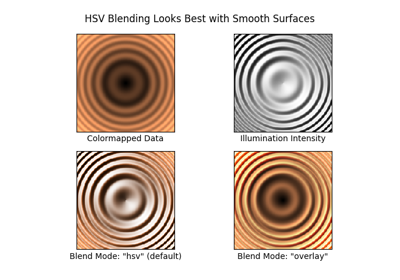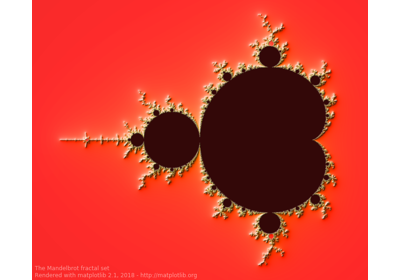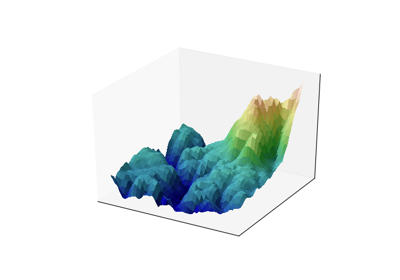Frontpage 3D example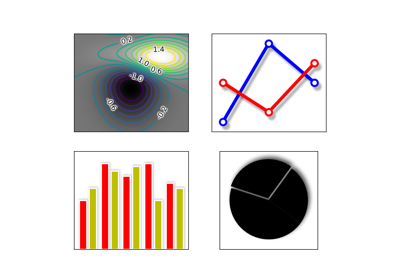Demo Agg Filter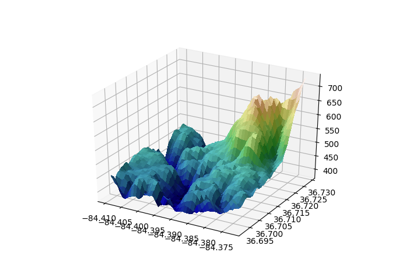Custom hillshading in a 3D surface plot